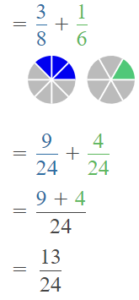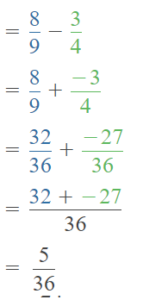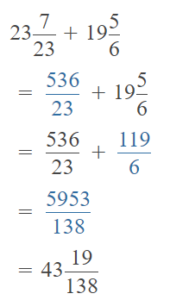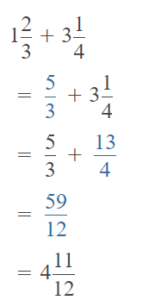# Math in Focus Grade 5 Chapter 3 Practice 7 Answer Key Real-World Problems: Fractions and Mixed Numbers

Go through the Math in Focus Grade 5 Workbook Answer Key Chapter 3 Practice 7 Real-World Problems: Fractions and Mixed Numbers to finish your assignments.

## Math in Focus Grade 5 Chapter 3 Practice 7 Answer Key Real-World Problems: Fractions and Mixed Numbers

Question 1.
Elena has 1 2 pieces of banana bread. She gives an equal amount of banana bread to 5 friends. How many pieces of banana bread does she give each friend?
2$$\frac{2}{5}$$ pieces of banana bread that she give each friend
Explanation:
Elena has 1 2 pieces of banana bread.
She gives an equal amount of banana bread to 5 friends.
12 is shared between 5 friends
12 ÷ 5
$$\frac{12}{5}$$ =
$$\frac{10}{5}$$ + $$\frac{2}{5}$$
= 2 + $$\frac{2}{5}$$
2$$\frac{2}{5}$$ pieces of banana bread that she give each friend

Question 2.
A utility bill shows that a household used 2,001 gallons of water in a 5-day period. What was the average amount of water used by the household each day?
400 gallons
Explanation:
A utility bill shows that a household used 2,001 gallons of water in a 5-day period.
2000 ÷  5 = 400
400 gallons was the average amount of water used by the household each day

Question 3.
A ball of string is 50 yards long. A shipper uses 5 yards of string to tie packages. The remaining string is then cut into 7 equal pieces. What is the length of each of the 7 pieces of string?
50 – 5 = 45
45 ÷  7 = 6.4
the length of each of the 7 pieces of string = 6.4
Explanation:
A ball of string is 50 yards long.
A shipper uses 5 yards of string to tie packages.
50 – 5 = 45
The remaining string is then cut into 7 equal pieces.
7 x 6 = 42
42 is the length of each of the 7 pieces of string

Question 4.
Steve picks 55 pounds of pears. He packs an equal amount of pears into 6 bags. He then has 4 pounds of pears left. What is the weight of pears in each bag?
10.1  is the weight of pears in each bag
Explanation:
Steve picks 55 pounds of pears.
He packs an equal amount of pears into 6 bags.
55 ÷  6 = 6.1
9 x 6 = 54
He then has 4 pounds of pears left.
6.1 + 4 = 10.1
10.1  is the weight of pears in each bag

Question 5.
Jeremy puts an empty container under a leaking faucet. In the first hour, $$\frac{3}{8}$$ quart of water collects. In the second hour, $$\frac{1}{6}$$ quart of water collects. How much water collects in the container in the two hours?
$$\frac{13}{24}$$ water collects in the container in the two hours
Explanation:
Jeremy puts an empty container under a leaking faucet.
In the first hour, $$\frac{3}{8}$$ quart of water collects.
In the second hour, $$\frac{1}{6}$$ quart of water collects.
$$\frac{3}{8}$$ + $$\frac{1}{6}$$$$\frac{13}{24}$$ water collects in the container in the two hours

Question 6.
Arnold buys $$\frac{8}{9}$$ pound of ground turkey. He uses $$\frac{3}{4}$$ pound or the ground turkey to make meatballs. How many pounds of ground turkey are left?
$$\frac{5}{36}$$
Explanation:
Arnold buys $$\frac{8}{9}$$ pound of ground turkey.
He uses $$\frac{3}{4}$$ pound or the ground turkey to make meatballs.
$$\frac{8}{9}$$ – $$\frac{3}{4}$$ = $$\frac{5}{36}$$$$\frac{5}{36}$$ pounds of ground turkey are left

Question 7.
A snail is at the bottom of a well. In the first 1 0 minutes, the snail climbs 23$$\frac{7}{23}$$ inches. in the next 10 minutes, it climbs 19$$\frac{5}{6}$$ inches. How far is the snail from the bottom of the well after 20 minutes?
43$$\frac{19}{138}$$
Explanation:
A snail is at the bottom of a well. In the first 1 0 minutes, the snail climbs 23$$\frac{7}{23}$$ inches.
in the next 10 minutes, it climbs 19$$\frac{5}{6}$$ inches.
23$$\frac{7}{23}$$ + 19$$\frac{5}{6}$$ = 43$$\frac{19}{138}$$43$$\frac{19}{138}$$ far is the snail from the bottom of the well after 20 minutes

Johnny is jogging along a track. He has already jogged 1$$\frac{2}{3}$$ miles. He plans to jog a total of 3$$\frac{1}{4}$$ miles. How many miles does he have left to jog?
4$$\frac{11}{12}$$
He has already jogged 1$$\frac{2}{3}$$ miles.
He plans to jog a total of 3$$\frac{1}{4}$$ miles.4$$\frac{11}{12}$$ miles that he have left to jog